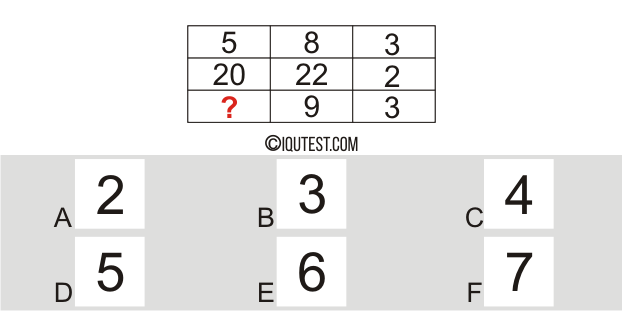# IQ Test Numerical [Number Matrices]

This test is to measure your logical and math skills in answering test questions according to the patterns in each question.

Find the correct answer number on each question of this test according to the pattern of numbers on each line.

Example Question:
Find The Missing Number?Explanation:

Numerical Test Number Matrices
• 20 questions
• Numerical, Logical, Mathematical
• Time: 20 minutes

## More Challenging Tests